# Taylor Series Using Recursion in C

## Taylor Series Using Recursion in C Language with Examples:

In this article, I am going to discuss Taylor Series using recursion in C Language with Examples. Please read our previous article, where we discussed the Power of a given number by using recursion in C Language with Examples.

##### Understanding Taylor Series

Before jumping to coding stuff, we have to first calculate our recursive function formula for Taylor Series. Let’s have a look at the Below expression which is the mathematical expression of the Taylor Series.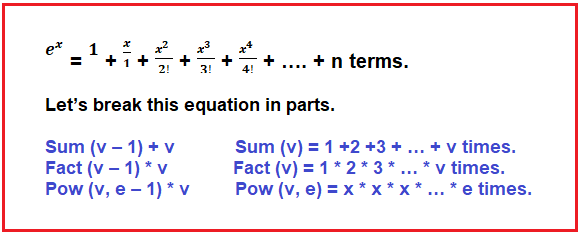These are the three steps that all of them together make the Taylor Series. Taylor Series is nothing but Sum between the result of (Pow / Fact). Let’s review our previous examples with the help of a diagram.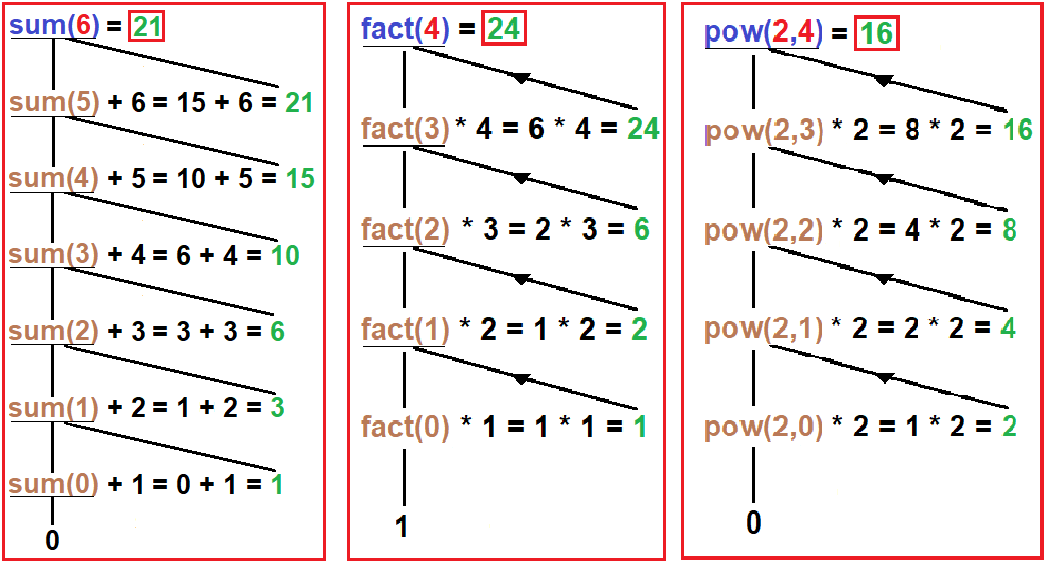In the above diagram, we are showing the Tracing Tree of Sum, Fact, and Pow function which we discussed in our previous three articles. If you haven’t read them, then first check out the previous examples as there we explain them in brief explanation. Now, let’s jump on the code part.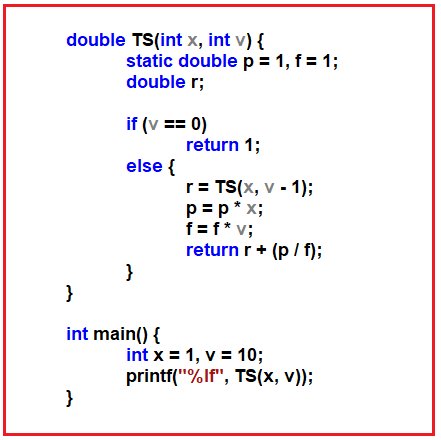In the above code, we create a function named TS (Taylor Series). This function requires two parameters, the first parameter holds the value of power we want to calculate e, and the second parameter holds the number of n terms in the Taylor Series as we have more terms, we will get a more accurate result.

In the function, we create two variables of type static double because we want to preserve the last value of these two variables that’s why we declared them as static.

Next, we define our base condition which is if v == 0 then return 1. In the else part we initialize our r variable to store the result of the recursive call. Here also we modified the value of p and f to calculate the power to x and factorial to v. we will understand it in more detail with a diagram explanation.

Next, it will return r + (p / f) which holds our previous call result and will use in unfinished calls. And as the final result will calculate then it returns that value inside the main function where we call it. And finally, it prints the value on the screen. Now let’s jump on to the Tracing part.

##### Tracing Tree of the above Taylor Series Program:

Now, Let’s jump on the Tracing Tree where we will understand each and every step.

##### Step1: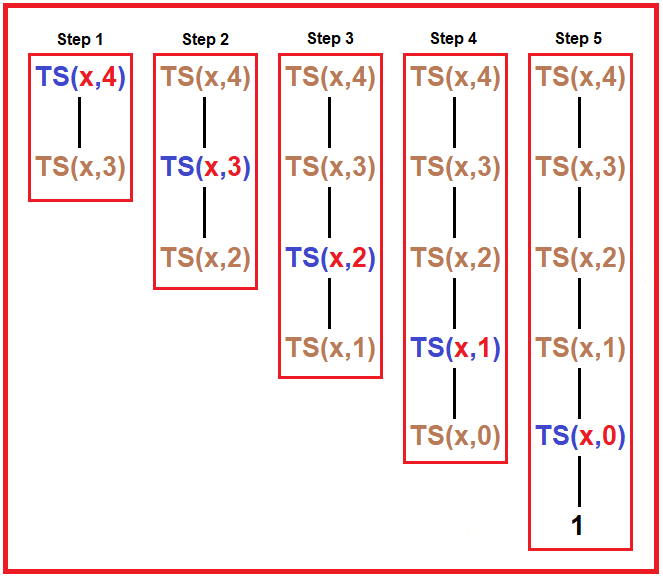Tree – In the above diagram, there are 5 steps. In every step, we have shown every recursive call of the TS function. As shown in the diagram, we passed x (it is a number but here we just use x for explanation) and 4 as a parameter.

1. In 1st step, it checks if 4 == 0? No, then it goes to the else part where we initialized the r variable which holds the result of TS (x, 4 – 1), and the next 3 steps after the call will perform on the returning time from function calls.
2. In 2nd step, it checks if 3 == 0? No, then it goes to the else part where we initialized the r variable which holds the result of TS (x, 3 – 1), and the next 3 steps after the call will perform on the returning time from function calls.
3. In 3rd step, it checks if 2 == 0? No, then it goes to the else part where we initialized the r variable which holds the result of TS (x, 2 – 1), and the next 3 steps after the call will perform on the returning time from function calls.
4. In 4th step, it checks if 1 == 0? No, then it goes to the else part where we initialized the r variable which holds the result of TS (x, 1 – 1), and the next 3 steps after the call will perform on the returning time from function calls.
5. In 5th step, it checks if 0 == 0? Yes, then it returns 1. And from here the function starts to perform the next 3 steps, 1 will store in the r variable and then it goes to the next statement of TS (x, 1).
##### Step2: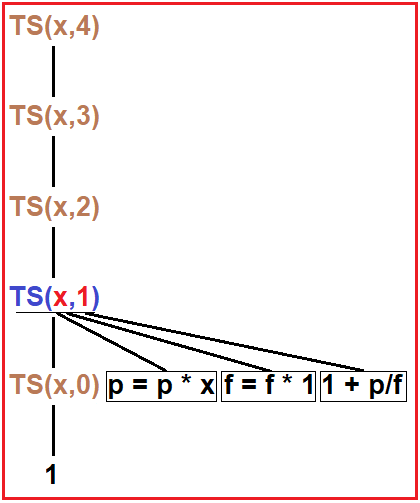Tree – As we declare p and f as the static double of default value 1. In the above diagram, the r variable holds the value 1, on the next lines, it will calculate p and f as –

p = p * x

f = f * 1

here we modify both the variable and on the next line TS (x, 1) return r + (p / f) to TS (x, 2) in r variable.

##### Step3: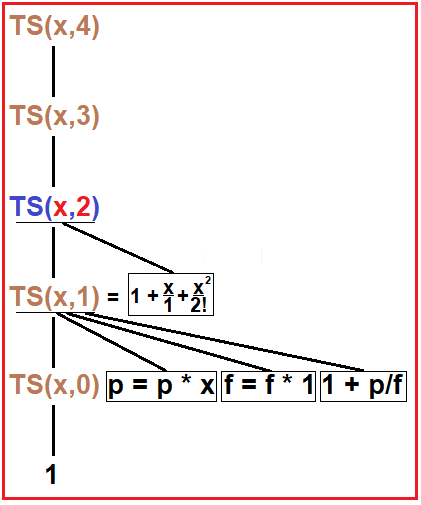Tree – In TS (x,2), Again we will modify p and f as –

p = p * x * x

f = f * 1 * 2

and then TS (x, 2) return r + (p / f) to TS (x, 3) in r variable.

##### Step4: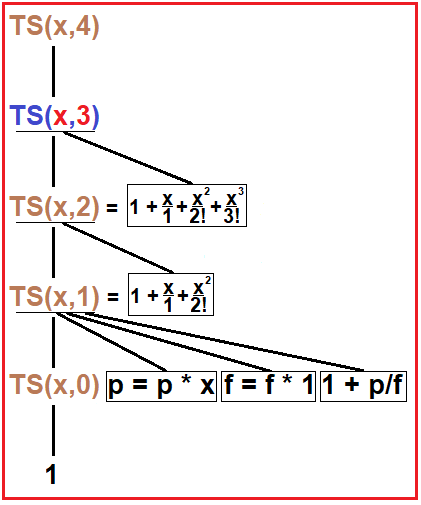Tree – In TS (x,3), Again we will modify p and f as –

p = p * x * x * x

f = f * 1 * 2 * 3

and then TS (x, 3) return r + (p / f) to TS (x, 4) in r variable.

##### Step5: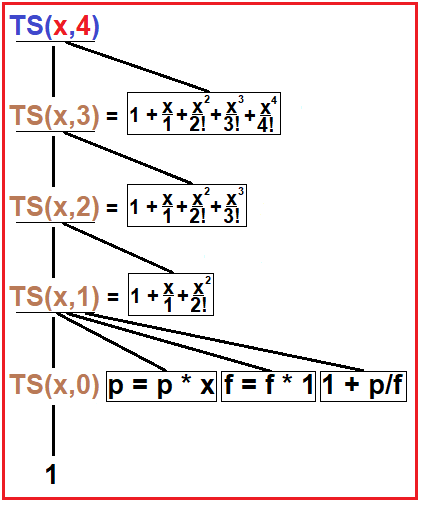Tree – In TS (x,4), Again we will modify p and f as –

p = p * x * x * x * x

f = f * 1 * 2 * 3 * 4

and then TS (x, 4) return r + (p / f) where we call this function inside our main function.

Now, let’s have a loop on some inputs and outputs –

TS (1, 4) – Output: 2.708333

TS (1, 6) – Output: 2.718056

TS (1, 10) – Output: 2.718282

We can clearly see in above outputs that as we increase the value of v in TS (x, v) function then we will get more precise result i.e. v: 4, 6, 10 and outputs: 2.708333, 2.718056, 2.718282

e2 -> TS (2, 10) – Output: 7.388995

e3 -> TS (3, 10) – Output: 20.079665

e4 -> TS (4, 10) – Output: 54.443104

##### Complete Code:
```#include <stdio.h>
double TS(int x, int v) {
static double p = 1, f = 1;
double r;

if (v == 0)
return 1;
else {
r = TS(x, v - 1);
p = p * x;
f = f * v;
return r + (p / f);
}
}

int main() {
int x = 1, v = 10;
printf("%lf", TS(x, v));
}
```

Output: 2.718282

In the next article, I am going to discuss Taylor Series using Horner’s Rule in C Language with Example. Here, in this article, I try to explain Taylor Series using Recursion in C Language with Example and I hope you enjoy this Taylor Series using Recursion in C Language with Example article.